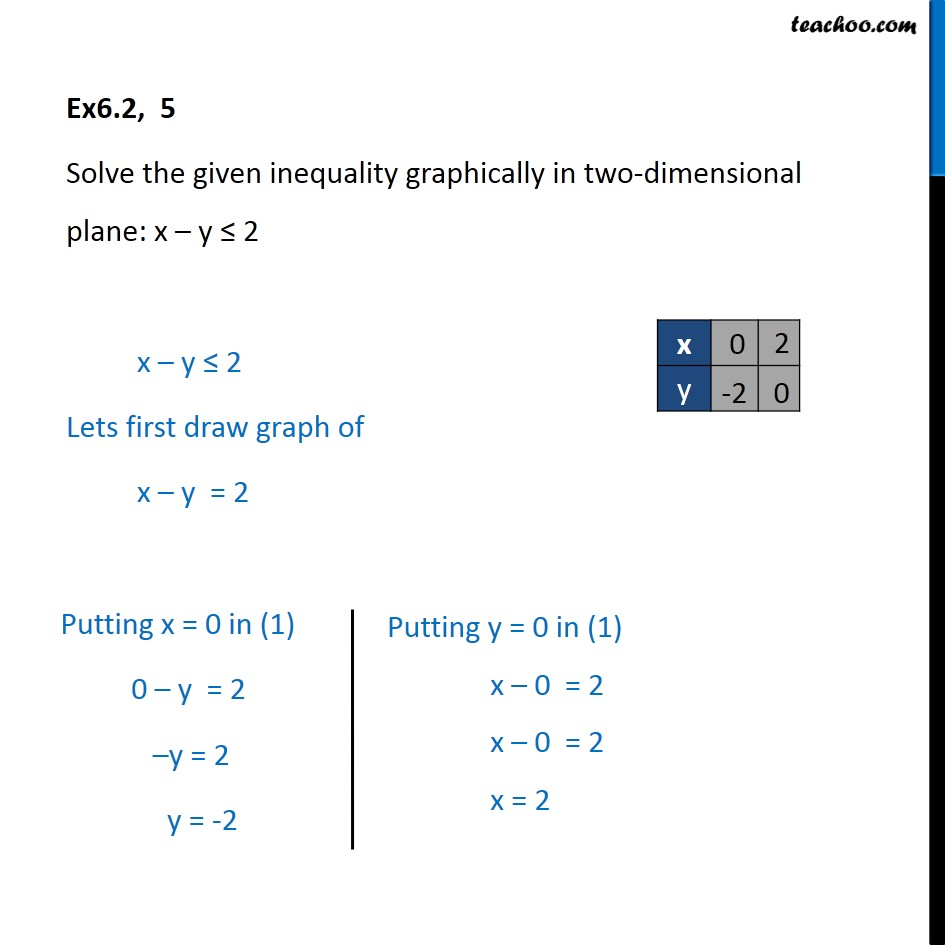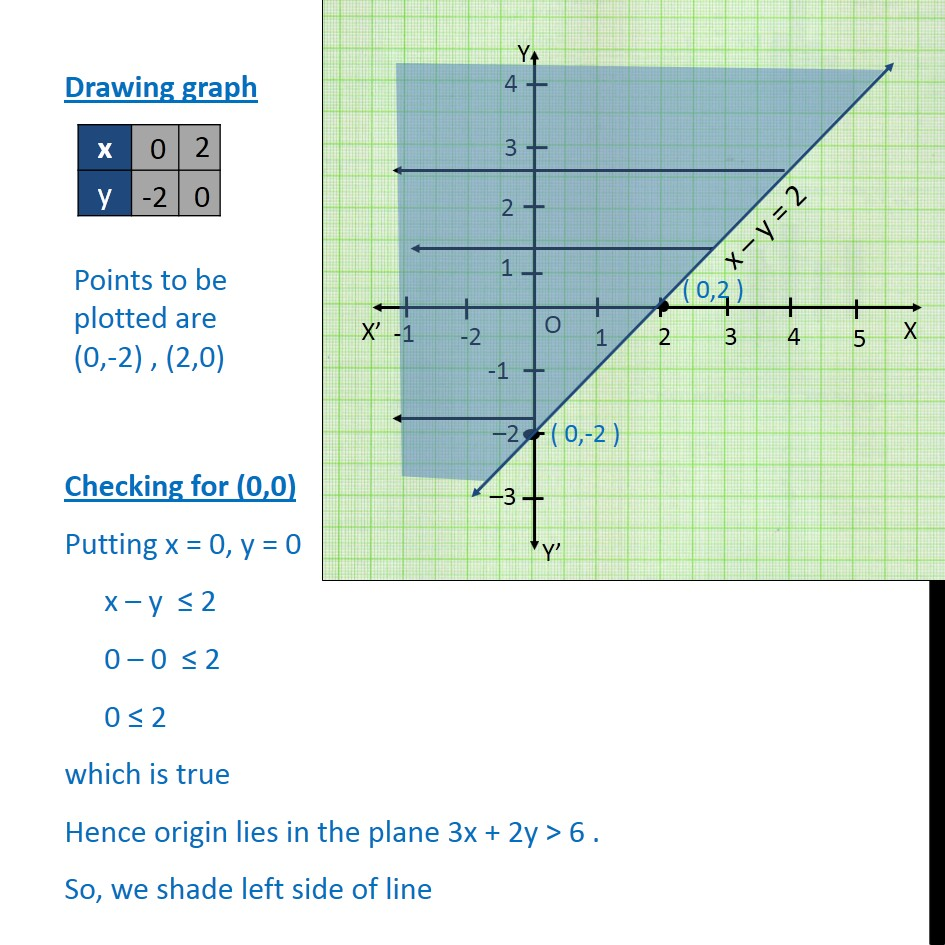Ex 6.2

Chapter 6 Class 11 Linear Inequalities (Term 2)
Serial order wise641 students have Teachoo Black. What are you waiting for?

### Transcript

Ex6.2, 5 Solve the given inequality graphically in two-dimensional plane: x – y ≤ 2 x – y ≤ 2 Lets first draw graph of x – y = 2 Drawing graph Checking for (0,0) Putting x = 0, y = 0 x – y ≤ 2 0 – 0 ≤ 2 0 ≤ 2 which is true Hence origin lies in the plane 3x + 2y > 6 . So, we shade left side of line